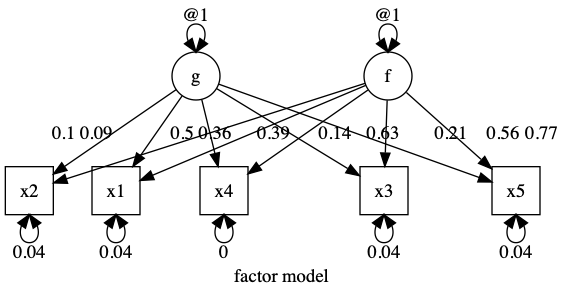# umx!

## EFA (Exploratory factor analysis) using umx

Factor analysis takes a number of measured variables (manifest variables) and accounts for these in terms of a (smaller) number of latent variables, and uncorrelated residual variance, specific to each manifest variable. The number of factors to retain can be estimated using various methods including Horn’s parallel analysis. These factors define a multi-dimensional space within which the items are located. The factors themselves can be transformed (rotated) to a simpler structure.

Performing a factor analysis in R is straightforward, as long as one does not have missing data. This code snippet gives an example of an analysis of 5 variables from the ubiquitous `mtcars` data set, retaining 2-factors:

``````
factanal(~ mpg + disp + hp + wt + qsec, factors = 2, rotation="promax", data = mtcars)
#      Factor1 Factor2
# mpg  -0.851   0.345
# disp  0.871  -0.356
# hp    0.621  -0.701
# wt    0.996
# qsec -0.123   0.906
``````

In `umx`, we can replicate this analysis with the `umxEFA` function:

``````vars = c("mpg", "disp", "hp", "wt", "qsec")
m2 = umxEFA(name = "test", factors = 2, data = mtcars[, vars])
x = mxStandardizeRAMpaths(m2, SE= TRUE)
``````

``````load = m2\$A\$values[manifests, latents]

if rotation == "varimax"){
} else if (rotaions == "promax"){
}

``````

require(umx)

manifests = names(data) m1 = umxRAM(model = name, data = data, autoRun = FALSE, umxPath(factors, to = manifests, connect = “unique.bivariate”), umxPath(v.m. = manifests), umxPath(v1m0 = factors, fixedAt = 1) )

data(demoOneFactor) latents = c(“g”, “f”) manifests = names(demoOneFactor) myData = mxData(cov(demoOneFactor), type = “cov”, numObs = 500) m1 = umxRAM(“f”, data = myData, umxPath(latents, to = manifests, connect = “unique.bivariate”), umxPath(var = manifests), umxPath(var = latents, fixedAt = 1) )

plot(m1, splines = FALSE)

```

|name | Estimate| SE| |:———-|——–:|—–:| |g_to_x1 | 0.090| 2.759| |g_to_x2 | 0.101| 3.535| |g_to_x3 | 0.139| 3.992| |g_to_x4 | 0.360| 4.523| |g_to_x5 | 0.207| 5.456| |f_to_x1 | 0.387| 0.639| |f_to_x2 | 0.496| 0.717| |f_to_x3 | 0.560| 0.991| |f_to_x4 | 0.634| 2.569| |f_to_x5 | 0.765| 1.474| |x1_with_x1 | 0.040| 0.003| |x2_with_x2 | 0.035| 0.003| |x3_with_x3 | 0.040| 0.003| |x4_with_x4 | 0.000| 0.071| |x5_with_x5 | 0.040| 0.005| |g_with_g | 1.000| 0.000| |f_with_f | 1.000| 0.000|  “χ²(0) = 1.85, p = 1.000; CFI = 1; TLI = 1; RMSEA = 0”